# nth Degree Polynomial

nth Degree Polynomial

A polynomial is the parent term used to describe a certain type of algebraic expressions that contain variables, constants, and involve the operations of addition, subtraction, multiplication, and division along with only positive powers associated with the variables.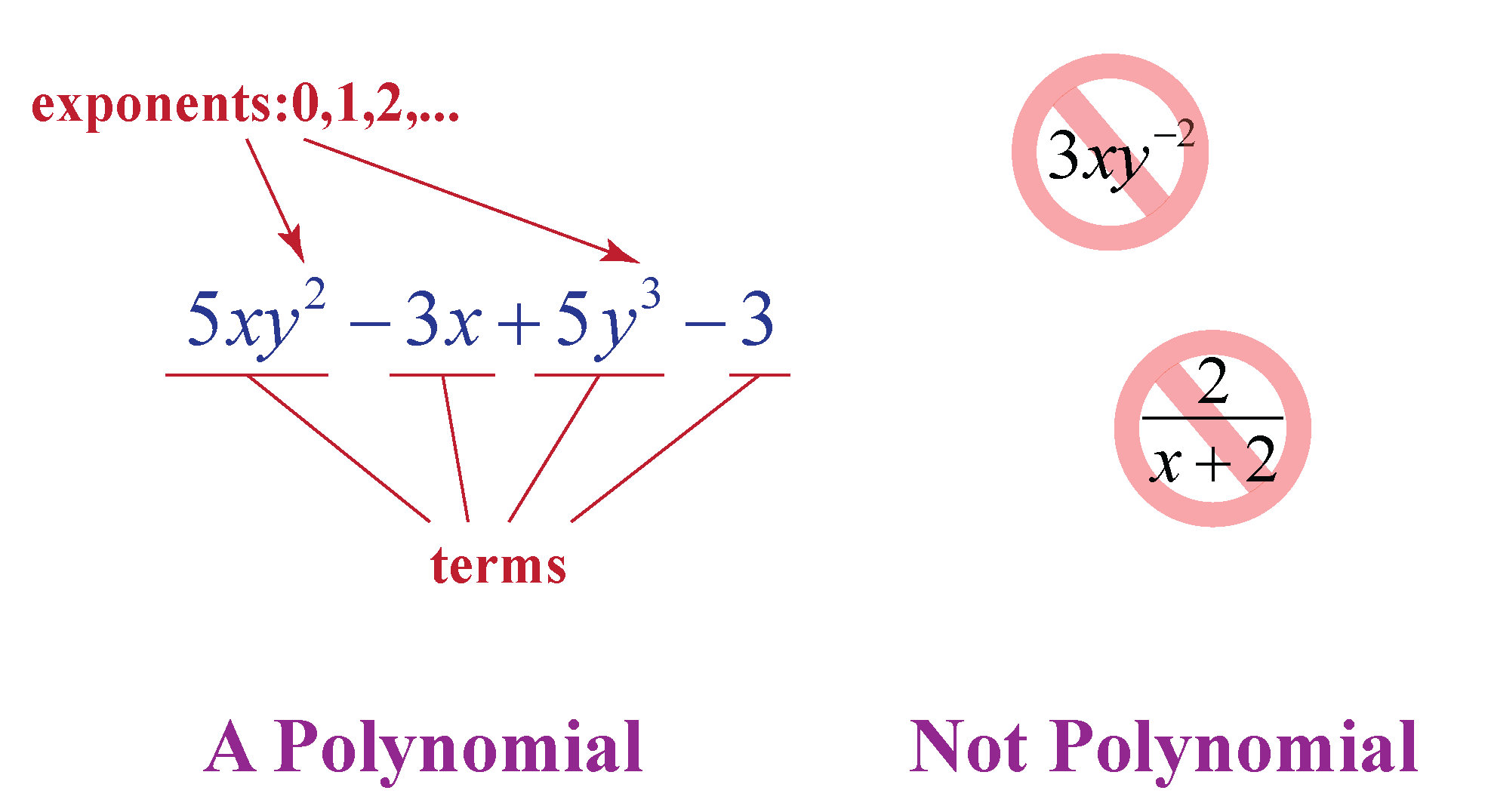In this mini-lesson, we will study about the nth degree polynomials using nth degree polynomial definition and nth degree polynomial examples.

Check-out interactive examples on nth degree polynomial function with real coefficients. Do try your hand at a few practice questions at the end of the page.

## Lesson Plan

 1 What Do You Mean by Nth Degree Polynomials? 2 Important Notes on Nth Degree Polynomials 3 Challenging Questions on Nth Degree Polynomials 4 Solved Examples on Nth Degree Polynomials 6 Interactive Questions on Nth Degree Polynomials

## What Do You Mean by Nth Degree Polynomials?

### Nth Degree Polynomials: Definition

The degree of a polynomial is defined as the highest power of the variable in the polynomial.

Thus, Nth degree polynomial is any polynomial with the highest power of the variable as $$n$$.

This means that any polynomial of the form:

$$P(x) = a_n x^n + a_{n-1} x^{n-1} + a_{n-2} x^{n-2} + .... + a_0$$

is an nth degree polynomial function with real coefficients and the variable is represented as $$x$$, having highest power $$n$$.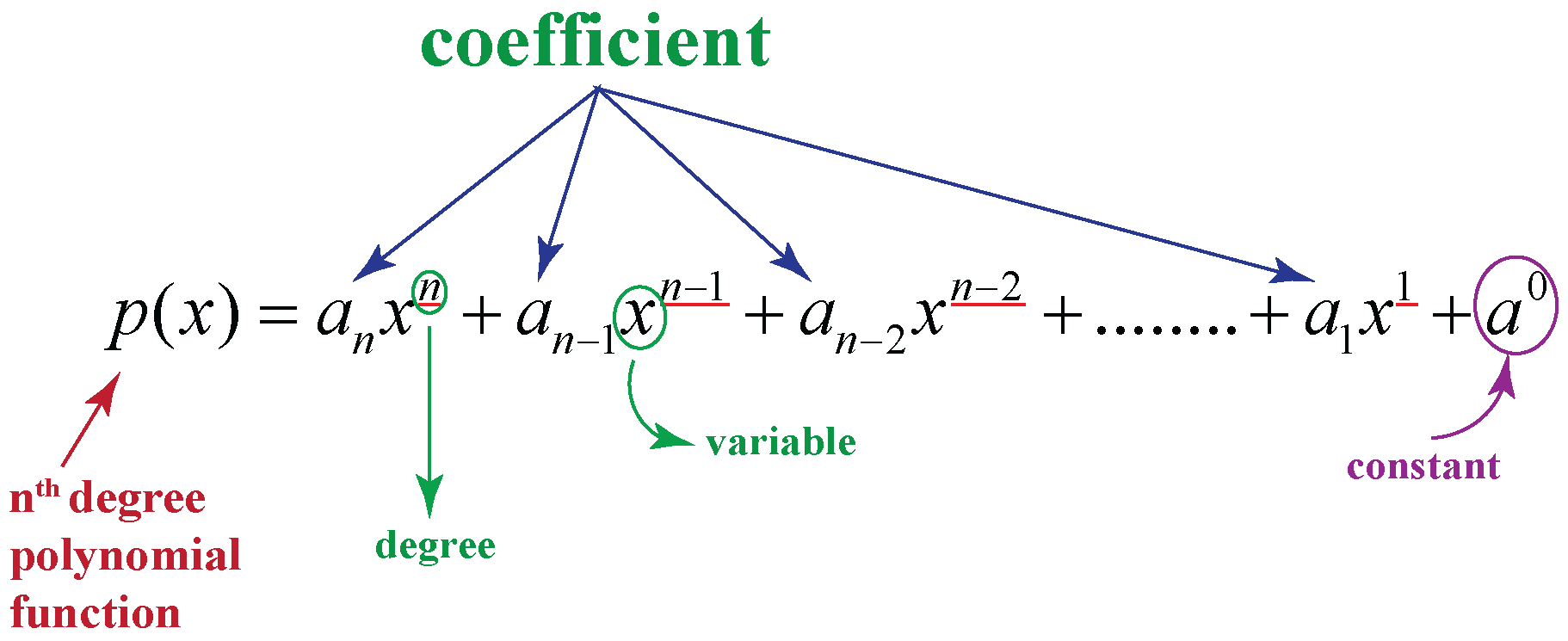$$n$$ will take all whole number values.

For example: Expressions, like $$\sqrt{x} + 2x + 7$$, are not a polynomial because all the powers of variable $$x$$ are not whole numbers.

$$a_n, a_{n-1}, a_{n-2}, ..., a_0$$ are the coefficients that take numerical values as their inputs.

This is also the generalized way to represent different types of polynomials, i.e., coefficients $$a_n, a_{n-1}, a_{n-2}, ..., a_0$$ and the power $$n$$ can have numerical values depending upon the types of polynomials they represent.

### Nth Degree Polynomials: Examples

Cubic polynomial: A cubic polynomial can be generally interpreted as a form of nth degree polynomial with the value of $$n$$ as 3: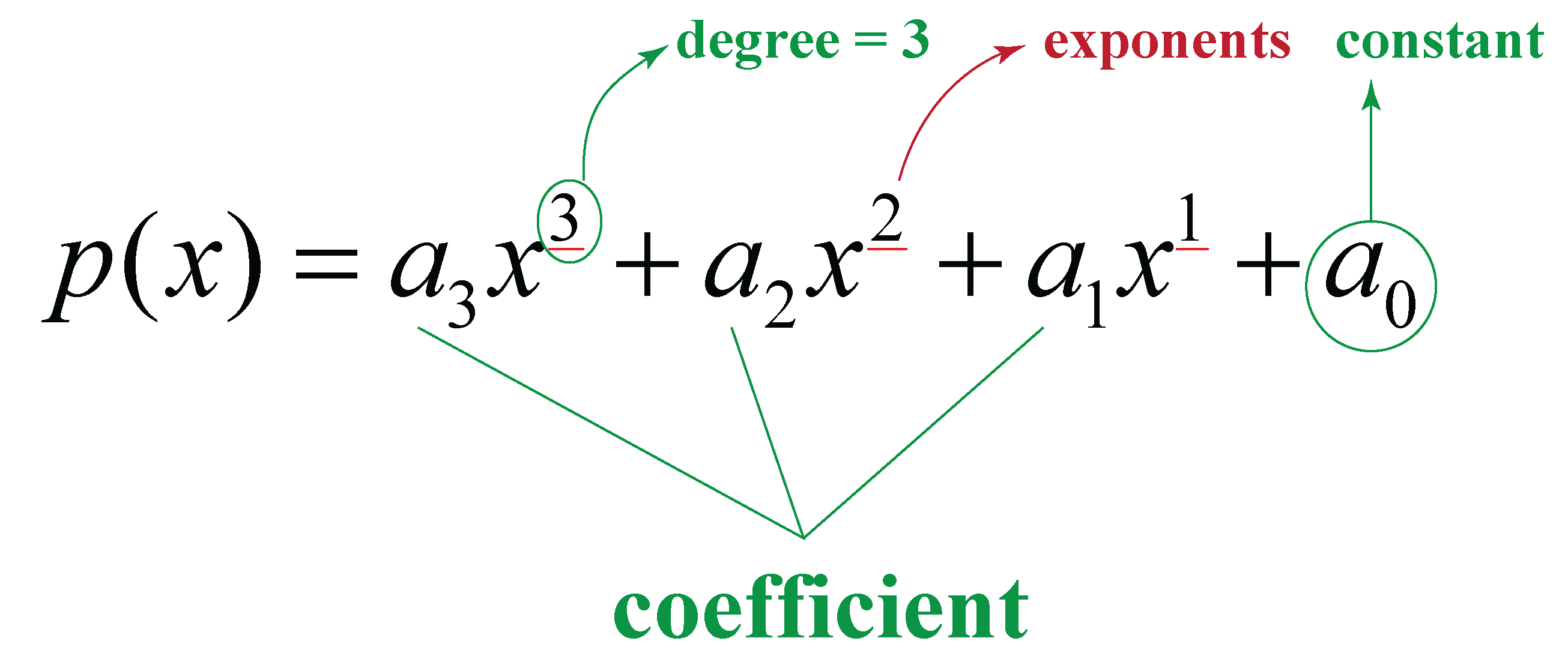Quadratic polynomial: A quadratic polynomial can be generally interpreted as a form of nth degree polynomial with the value of $$n$$ as 2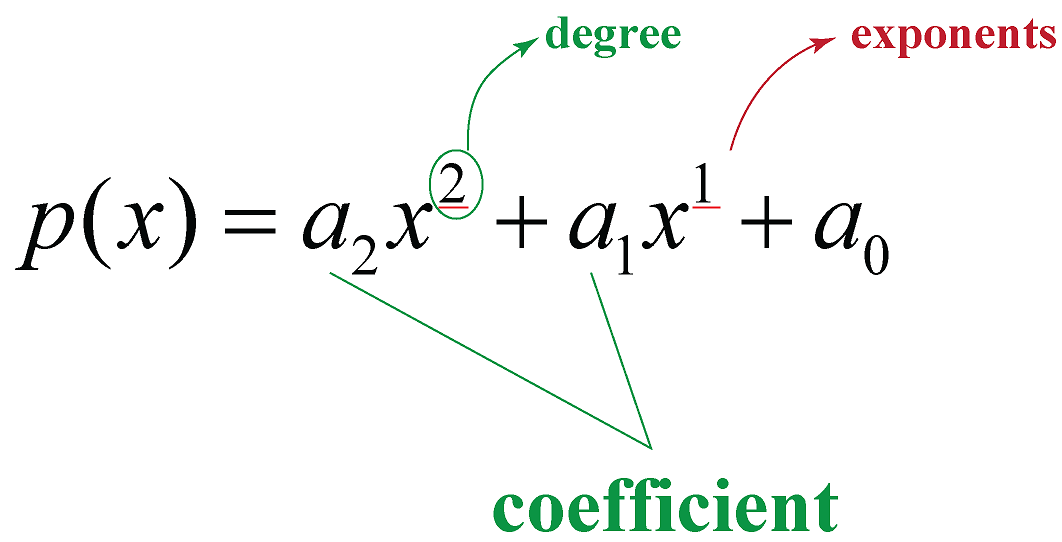## General Form of Nth Degree Polynomial

The general form used to represent nth degree polynomial is,

$$P(x) = a_n x^n + a_{n-1} x^{n-1} + a_{n-2} x^{n-2} + .... + a_0$$Here, $$a_0, a_1, a_2, ..., a_n$$ are the coefficients that take numerical values as their inputs, $$x$$ is the variable, and $$n$$ is the degree of the polynomial, which is a whole number.

 General form: $$P(x)$$ = $$a_n x^n + a_{n-1} x^{n-1} + a_{n-2} x^{n-2} + .... + a_0$$

The following table shows few nth degree polynomials for different values of $$n$$,

Polynomial Degree( Values of n) Example
Zero Polynomial not defined $$P(x) = 0$$
Non-Zero Constant 0 $$P(x) = 5$$
Linear Polynomial 1 $$P(x)$$ = $$7x + 2$$
Quadratic Polynomial 2 $$P(x)$$ = $$x^2 - x + 5$$
Cubic Polynomial 3 $$P(x)$$ = $$2x^3 + 3x^2 + x - 3$$
Quartic Polynomial 4 $$P(x)$$= $$3x^4 + 2x^3 + x^2 - x + 8$$
Quintic Polynomial 5 $$P(x)$$ = $$4x^5 + x^3 + x^2 - x$$
Hexic Polynomial 6 $$P(x)$$ =$$x^6 + x^3 - x$$
Septic/Heptic Polynomial 7 $$P(x)$$ = $$x^7 - x^6 - x$$
Octic Polynomial 8 $$P(x)$$ = $$x^8 + x^7 - x^5 + x^3$$
Nonic Polynomial 9 $$P(x)$$ =$$x^9 + 4$$
Decic Polynomial 10 $$P(x)$$= $$x^{10} - 4x + 2$$

## Zeros of Nth Degree Polynomial

Zeros or roots of an nth degree polynomial are those values that make the value of polynomial as '0'.

For the given nth degree polynomial:

$$P(x) = a_n x^n + a_{n-1} x^{n-1} + a_{n-2} x^{n-2} + .... + a_0$$

Zeros are defined as those $$\alpha, \beta, ... , n$$ values which when replace $$x$$ in $$P(x)$$, would give '0'.

A polynomial of degree $$n$$ will have $$n$$ number of zeros.

Zeros of any polynomial can be found using its graphical representation. The number of times the graph intersects the x-axis is equal to the number of distinct zeros the polynomial has.

For example:

For the polynomial shown in the following graph: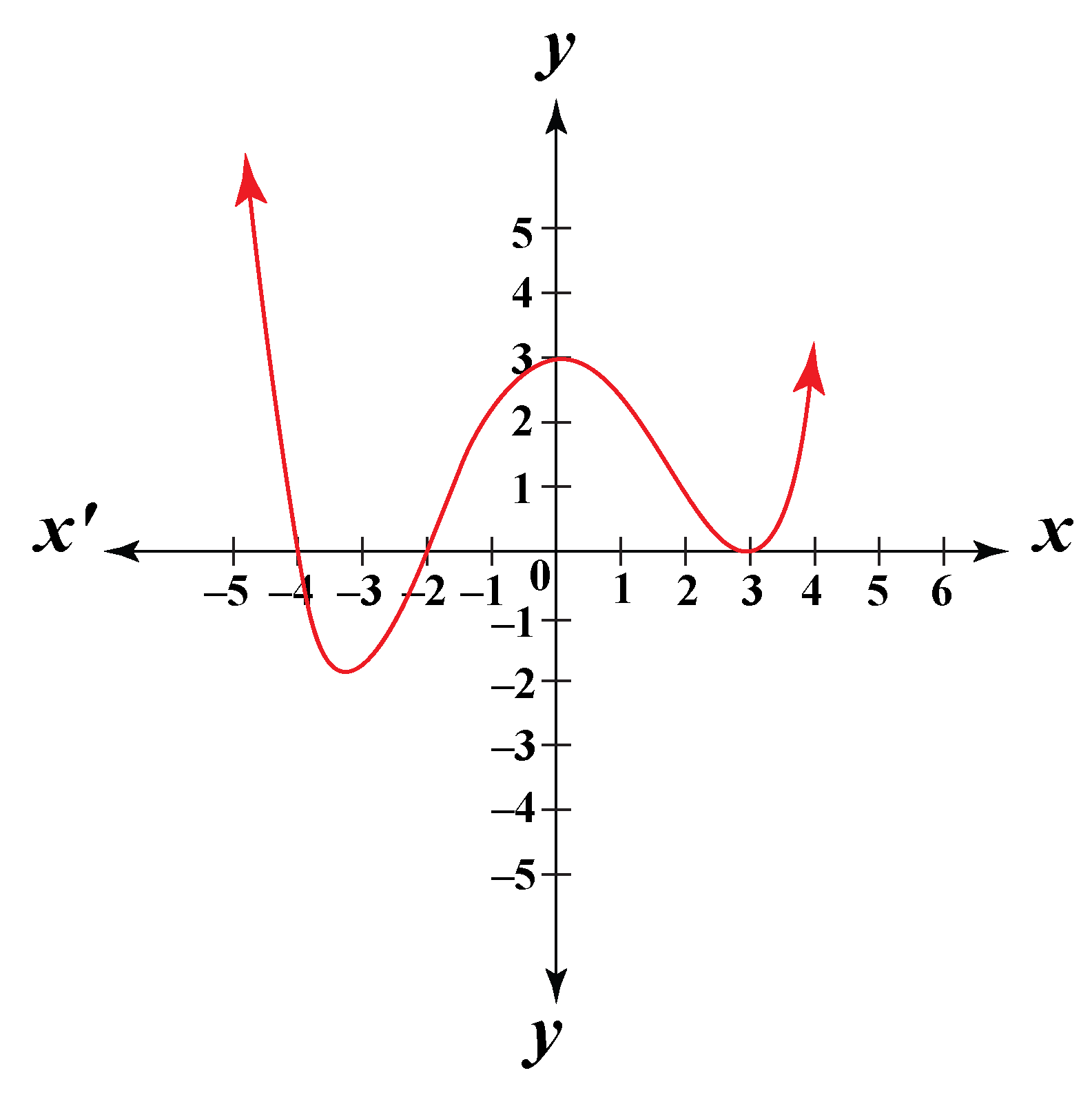Number of distinct zeros = number of intersections of the graph with the x-axis.

Here, the graph intersects the x-axis 3 times, which means that the polynomial will have 3 distinct zeros at $$x =$$ respectively.

## Solved Examples

 Example 1

Jennifer is solving questions on polynomials. Can you help her in finding the degree and zeros of the following polynomial,

$$x^2 - x - 6$$

Solution

For the given polynomial,

$$P(x) = x^2 - x - 6$$

We know,

Highest power of the variable $$x$$ = 2

Thus, the degree of the polynomial = 2

To find the zeros of the polynomial, we will make it a polynomial equation and them use factorization:

$$x^2 - x - 6 = 0$$

Using splitting the middle term method,

\begin{align*} x^2 - 3x + 2x - 6 &= 0 \\ x( x - 3) + 2( x - 3) &= 0 \\ x( x - 3) + 2( x - 3) &= 0 \end{align*}

Taking $$(x - 3 )$$ common, we get,

$$( x - 3)( x + 2) = 0$$

Hence, $$(x - 3)$$ and $$(x + 2)$$ are the factors of the given polynomial.

Equating the polynomial equation to 0, we get the zeros or roots of the polynomial.

\begin{align*} x &= 3 \\ x &= -2 \end{align*}

 $$\therefore$$ Degree of polynomial = 2 Zeros = 3, -2
 Example 2

For what value of $$k$$, -4 is the zero of the polynomial $$x^2 - 2x - (7k + 3)$$?

Solution

Zero of a polynomial $$P(x)$$ gives the value of polynomial as '0' when substituted for $$x$$.

Thus, for -4 to be the zero of the given polynomial,

\begin{align*} P(-4) = (-4)^2 - 2(-4) - (7p + 3 ) &= 0 \\ 16 + 8 -7k - 3 &= 0 \\ 7k &= 21 \\ k &= 3 \end{align*}

 $$\therefore$$ k = 3
 Example 3

Write the number of zeros of the polynomial, $$y = f(x)$$ in the following graph: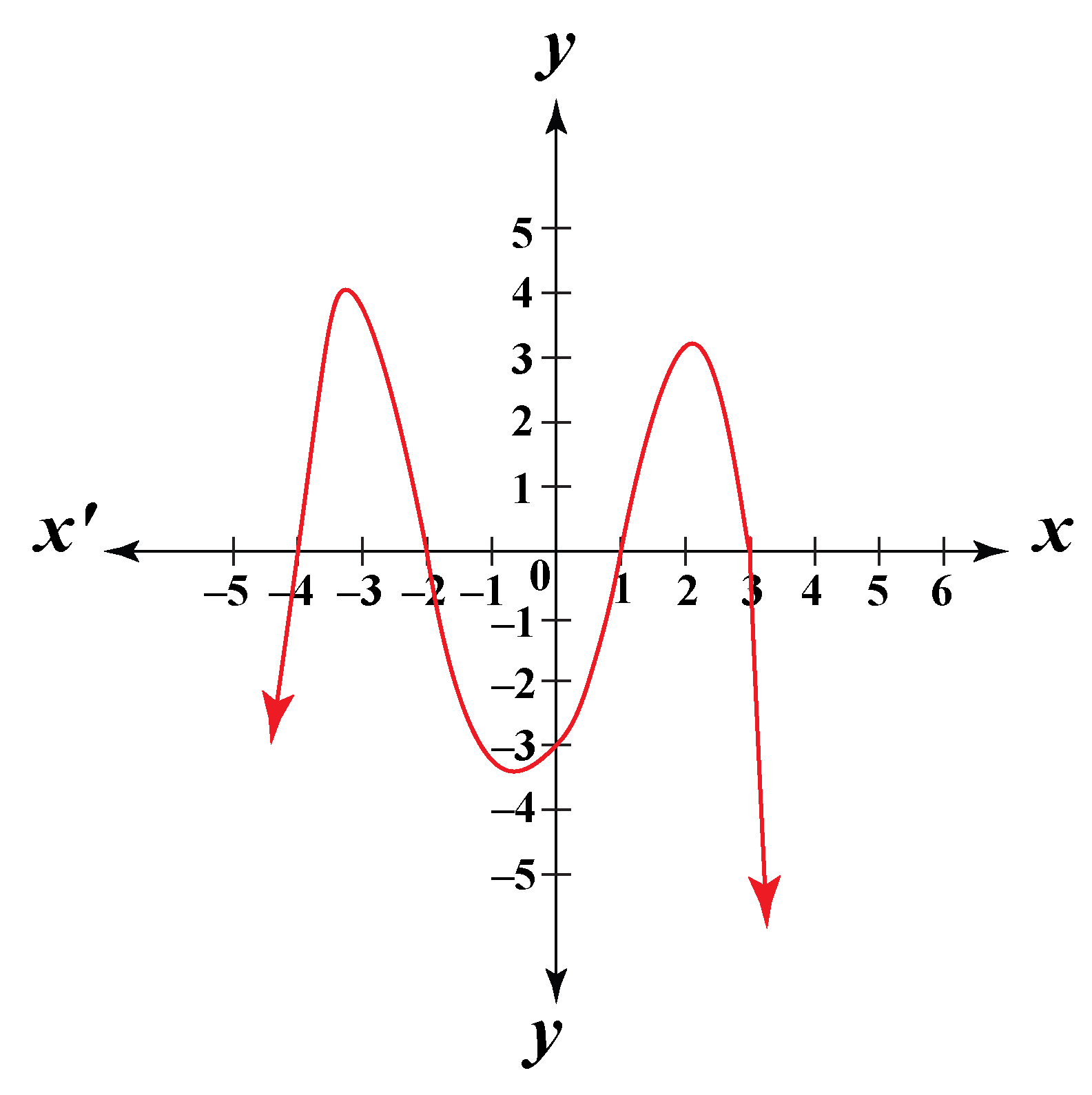Solution

For the given graph: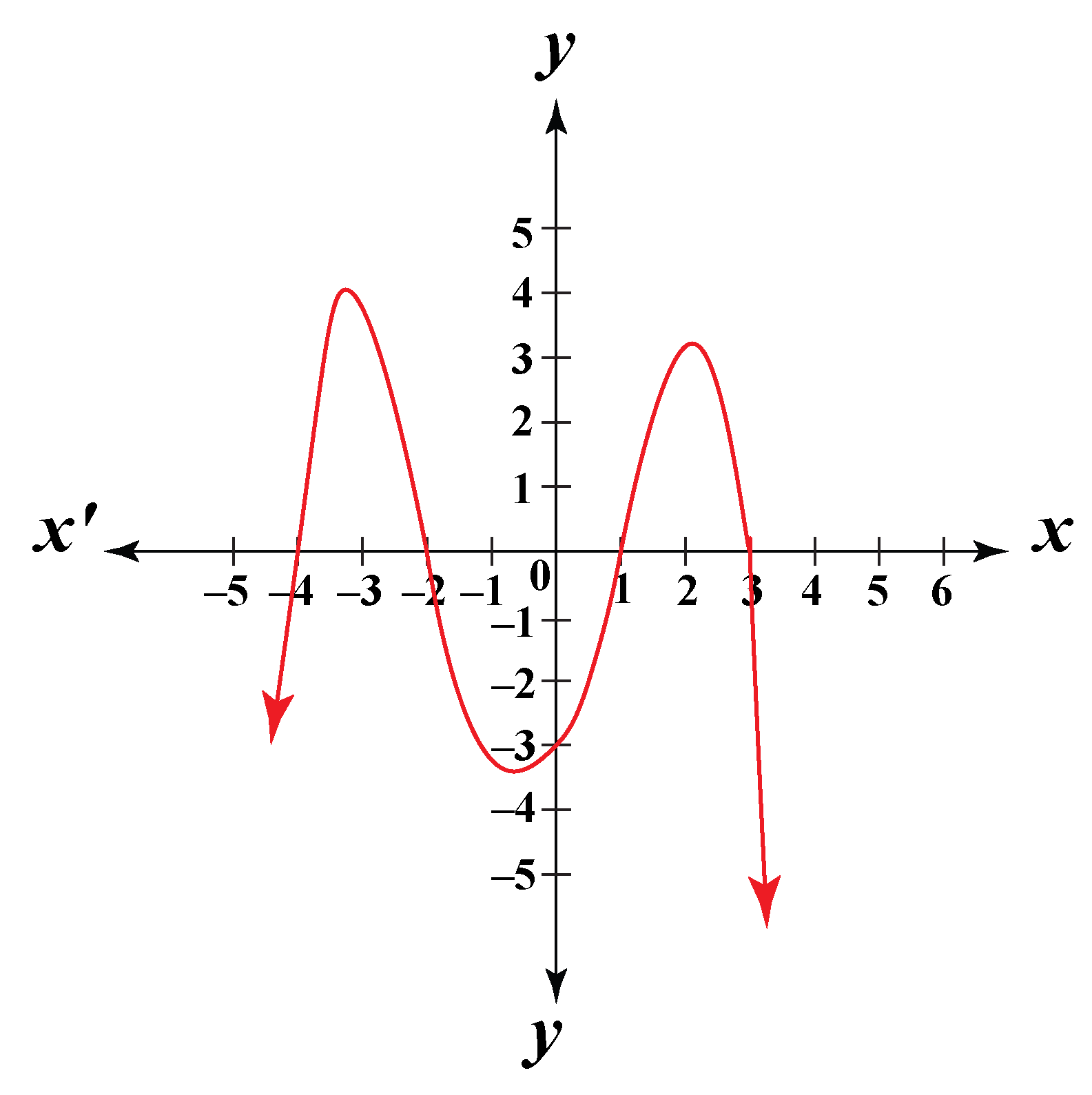The graph intersects the x-axis at 4 points, which means that for four different values of $$x$$, the polynomial will have a '0' value.

Thus, these four points will be the zeros of the given polynomial in the graph.

Number of zeros of the polynomial = 4

 $$\therefore$$ Number of zeros of the polynomial = 4Challenging Questions
• If one zero of the polynomial $$5x^2 + 13x – p$$ is reciprocal of the other, then find x.
• If the zeros of the polynomial $$f(x) = x^3 – 12x^2 + 39x + p$$ are in AP, find the value of p.

## Interactive Questions

Here are a few activities for you to practice. Select/Type your answer and click the "Check Answer" button to see the result.

## Let's Summarize

The mini-lesson targeted the fascinating concept of nth degree polynomial. The math journey around the nth degree polynomial started with what a student already knew and went on to creatively crafting a fresh concept in the young minds. Done in a way that not only it is relatable and easy to grasp, but also will stay with them forever.

At Cuemath, our team of math experts is dedicated to making learning fun for our favorite readers, the students!

Through an interactive and engaging learning-teaching-learning approach, the teachers explore all angles of a topic.

Be it worksheets, online classes, doubt sessions, or any other form of relation, it’s the logical thinking and smart learning approach that we, at Cuemath, believe in.

## FAQs on Nth Degree Polynomial

### 1.What does the nth degree mean?

The Nth degree of a polynomial means the degree of the polynomial or the highest power of the variable in the polynomial is $$n$$.

$$n$$ takes whole numbers as its values.

### 2.What is the degree in a polynomial?

The degree of a polynomial is defined as the highest power of the variable in the polynomial.

For example: Polynomial $$x^3 - 2x + 7$$ has degree 3

### 3.How do you find the degree n of a polynomial?

The degree of any polynomial is found by finding the highest power the variable in the polynomial has.

For example: The highest power of the variable $$x$$ in the polynomial $$P(x) = x^4 - 2x^2 + 7$$ is 4. Thus, it's degree is 4

### 4.How many zeros does a polynomial of degree n have?

The number of zeros of any polynomial is equal to the degree of the polynomial.

A polynomial of degree $$n$$ will have $$n$$ number of zeros.

### 5.What does nth mean?

Any generalized number is represented with $$n$$. In the case of nth degree polynomial, n represents the degree of the polynomial, that takes a whole number as its value.

### 6.How to solve nth degree polynomial?

The nth degree polynomial has degree $$n$$, which means that the highest power of the variable in the polynomial will be $$n$$.

Since, $$n$$ takes any whole number as its value, depending upon the type of equation, thus for different values of n, there are different types of equations, namely linear, quadratic, cubic, etc.

These different types of equations follow different methods to be solved for the value of the variable and zeros or roots.

For example:

i) Linear polynomial: To solve a linear polynomial, we directly equate the polynomial to '0' and find the zero or root of the polynomial.

ii)Quadratic polynomial: To solve a quadratic polynomial, we can use different methods, factorization, completing the square or the quadratic formula to calculate the zero or root.

### 7.What is the formula for a polynomial?

The general form used to represent nth degree polynomial is:

$$P(x) = a_0 x^n + a_1 x^{n-1} + a_2 x^{n-2} + .... + a_n$$

Here, $$a_0, a_1, a_2, ..., a_n$$ are the coefficients that take numerical values as their inputs, $$x$$ is the variable, and $$n$$ is the degree of the polynomial, which is a whole number.

### 8.What are the types of polynomials?

Polynomial can be categorized into different types based on the following parameters:

i) Degree of polynomial

• Degree -1 or $$infty$$: Zero polynomial
• Degree 0: Non zero constant
• Degree 1: Linear polynomial